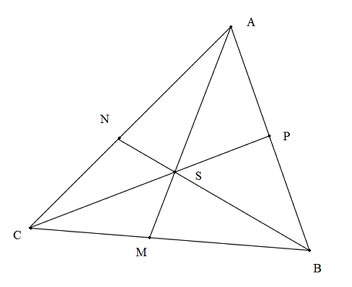# A cool geometry problem!

Geometry Level 3In the given figure,$S$ is the circumcentre of $\bigtriangleup ABC$.Then find the value of $\dfrac{R}{AM}+\dfrac{R}{BN}+\dfrac{R}{CP}$,where $R$ is the circumradius of the $\bigtriangleup ABC$.

×

Problem Loading...

Note Loading...

Set Loading...